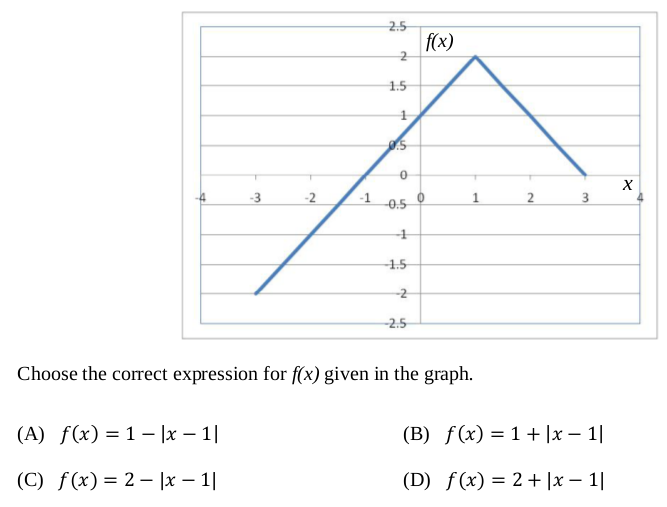# GATE | GATE-CS-2016 (Set 2) | Question 10

• Last Updated : 28 Jun, 2021

Choose the correct expression for f(x) given in the graph.(A) A
(B) B
(C) C
(D) D

Explanation: Simply, there are two test cases:

—> In graph, at x = 1, f(x) = 2

Let’s find the values at x = 1 in given options:

A) f(1) = 1 – |1-1| = 1 – 0 = 1
B) f(1) = 1 + |1-1| = 1 + 0 = 1
C) f(1) = 2 – |1-1| = 2 – 0 = 2
D) f(1) = 2 + |1-1| = 2 + 0 = 2

Hence (A) and (B) are incorrect.

Now,

—> In the graph, at x=0, f(x) = 1

Let’s find the values at x = 1 in the remaining options, i.e. C and D.

(c) f(0) = 2 – |0-1| = 2 – 1 = 1
(d) f(0) = 2 + |0-1| = 2 + 1 = 3

Hence, C is the correct answer.

This explanation is contributed by Mohit Gupta.

Quiz of this Question

My Personal Notes arrow_drop_up